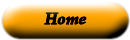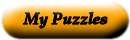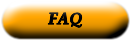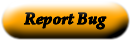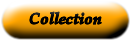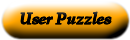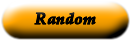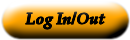### Geometry Vocabulary

 1 point _____ two angles in the same plane that have the same vertex and a common side but do not have any interior points in common 2 line _____ lines that meet to form right angles 3 ray _____ the numbers on a protractor used to measure angles 4 line segment _____ points not on the same line 5 plane _____ if three sides of one triangle are congruent to three sides of another triangle, then the two triangles are congruent 6 postulates _____ points on the same plane 7 noncollinear points _____ a line that intersects two or more other lines, each at a different point. 8 coplanar points _____ an angle that measures between 180 and 360 degrees 9 theorems _____ an angle that measures 360 degrees 10 straight angle _____ statements that can be proved 11 acute angle _____ in a right triangle, the sum of the squares of the lengths of the legs is equal to the square of the length of the hypotenuse 12 obtuse angle _____ angles that are interior angles on opposite sides of the transversal 13 transversal _____ statements accepted without proof 14 perpendicular _____ a triangle that has 3 sides all of different lengths 15 parallel _____ if point B is in the interior of angle AOC then the measure of angle AOB + the measure of angle BOC is equal to the measure of angle AOC 16 alternate interior angles _____ the two congruent sides of an isosceles triangle 17 same side interior angles _____ lines that are equal distance from each other and will never meet 18 corresponding angles _____ a tool used to measure angles 19 supplementary angles _____ if the square of the length of the longest side of a triangle is equal to the sum of the squares of the lengths of the two shorter sides, then the triangle is a right triangle 20 complementary angles _____ angles that are in the same position on the same side of a transversal. If you cut one out and layed it on top of the other they would match 21 protractor _____ a part of a line with one endpoint. It has an infinite number of points on it. 22 scales _____ 2 angles whose measurements together add up to 180 degrees 23 adjacent angles _____ in a right triangle, the side opposite the right angle 24 angle addition postulate _____ angle that measures between 90 and 180 degrees 25 complete angle _____ interior angles on the same side of the transversal 26 reflex angle _____ a triangle that has 3 sides all of which are the same length 27 zero angle _____ angle that measures between 0 and 90 degrees 28 isosceles triangle _____ part of a line with two endpoints. It has an infinite number of points on it. 29 scalene triangle _____ a triangle that has 2 sides with the same length 30 equilateral triangle _____ has no size and no dimensions; indicates a definite location 31 equiangular triangle _____ an angle that has a measurement of 0 degrees 32 legs _____ measures 180 degrees 33 acute triangle _____ a triangle with 3 acute angles 34 obtuse triangle _____ a triangle that has 3 angles all of which are the same measurement 35 side-side-side postulate _____ a triangle with one obtuse angle 36 side-angle-side postulate _____ if two angles and the included side of one triangle are congruent to corresponding parts of another triangle, the triangles are congruent 37 angle-side-angle postulate _____ a flat surface that has no thickness or boundaries 38 hypotenuse _____ if two sides and the included angle of one triangle are congruent to the corresponding parts of another triangle, then the triangles are congruent 39 pythagorean theorem _____ straight, but it has no thickness. It is an infinite set of points that extends in both directions. 40 converse of the Pythagorean theorem _____ 2 angles whose measurements together add up to 90 degrees

Use the "Printable HTML" button to get a clean page, in either HTML or PDF, that you can use your browser's print button to print. This page won't have buttons or ads, just your puzzle. The PDF format allows the web site to know how large a printer page is, and the fonts are scaled to fill the page. The PDF takes awhile to generate. Don't panic!Web armoredpenguin.com